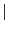Next: A robot kinematics example Up: Examples of applications of Previous: Examples of applications of   Contents

A geometrical example

The following example originated from the INRIA Geometrica project. It exemplifies that focusing on the problem at hand while keeping in view that interval analysis will be used allows one to develop a very efficient algorithm that is at the same time faster and safer (in term of quality of the result) than conventional approaches.

Consider 2 ellipses E1, E2 in the plane that are perfectly defined and the so-called bi-tangent i.e. the lines that are tangent to both ellipsis. There is 4 such lines but we assume that some criteria allows to choose two bi-tangents L1, L2. L1 intersects E1 at point P, E2 at and L2 intersects E1 at point R and E2 at point S. We construct now the vectors PQ, RS and then the determinant D=PQ RS. The problem is to determine the sign of D in a guaranteed manner (this sign is then used for another algorithm and a mistake in the sign has a very negative influence).

A classical approach to solve this problem is to consider that the 8 coordinates of the points P, Q, R, S are the unknowns, write 8 equations to determine these unknowns, solve the system and then determine the sign of D. But this approach has drawbacks:

• the system of equations is complex
• it is difficult to solve exactly
We may evidently use ALIAS to solve the system and uses the Newton procedure of ALIAS-Maple to compute the solution with a sufficient accuracy to state the sign of D. But this is overkill: we do not really want to determine the values of the coordinates of P, Q, R, S but only the sign of D.

Now we may note that the coordinates of the points are bounded as the points are included in the bounding box of the ellipses. Being given ranges for the unknowns we may compute the interval evaluation of the determinant. If the lower bound is positive or the upper bound is negative we may directly state the sign of the determinant. If not we bisect one the unknown coordinates: we has thus a list of boxes with 8 ranges in each box, one for each coordinate. For each box we check if the coordinates ranges allows the points P, Q, R, S to belong to the ellipsis and to the bi-tangents, otherwise we reject the box. Then we compute the interval evaluation of D: if the lower bound is positive we store the box in a special array of boxes, called the positive array and discard it from the list. Similarly if the upper bound is negative we store the box in the negative array and discard it from the list. When the list of boxes is exhausted we look at the number of elements in the positive and negative array: if one array has 0 element, then we have determined the sign of D. Otherwise we consider the array that has the lowest number of elements and restart the bisection procedure on this new list, eliminating boxes that do not describe points on the ellipsis or that are not on the bi-tangent. At the end of this process we may have eliminated all the boxes of the list or, in the worst case, we will have computed the coordinates of P, Q, R, S i.e. we will end up with the classical approach. Our test show however that this is seldom the case and the above procedure is much more faster than solving using the classical approach.

Still in some cases the sign of D cannot be safely computed: this may happen for example when some coordinates have very large values while other have very small one. Numerical round-off errors then prohibit the exact determination of the sign of D, a fact that is detected by interval analysis. But we may still in some cases solve the problem: for that purpose instead of using Cartesian coordinates we may use polar coordinates with different ranges for the unknowns in the determinant or we may use an extended arithmetics. In any case the algorithm is safe as it provides either a sign, which is guaranteed, or will state that the sign cannot be determined with the current arithmetics.Next: A robot kinematics example Up: Examples of applications of Previous: Examples of applications of   Contents
Jean-Pierre Merlet 2012-12-20